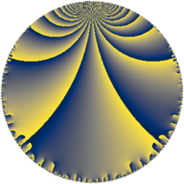# Properties

 Label 35.5.gLevel $35$ Weight $5$ Character orbit 35.g Rep. character $\chi_{35}(8,\cdot)$ Character field $\Q(\zeta_{4})$ Dimension $24$ Newform subspaces $1$ Sturm bound $20$ Trace bound $0$

# Related objects

## Defining parameters

 Level: $$N$$ $$=$$ $$35 = 5 \cdot 7$$ Weight: $$k$$ $$=$$ $$5$$ Character orbit: $$[\chi]$$ $$=$$ 35.g (of order $$4$$ and degree $$2$$) Character conductor: $$\operatorname{cond}(\chi)$$ $$=$$ $$5$$ Character field: $$\Q(i)$$ Newform subspaces: $$1$$ Sturm bound: $$20$$ Trace bound: $$0$$

## Dimensions

The following table gives the dimensions of various subspaces of $$M_{5}(35, [\chi])$$.

Total New Old
Modular forms 36 24 12
Cusp forms 28 24 4
Eisenstein series 8 0 8

## Trace form

 $$24 q + 20 q^{3} - 48 q^{5} + 72 q^{6} + O(q^{10})$$ $$24 q + 20 q^{3} - 48 q^{5} + 72 q^{6} - 112 q^{10} + 156 q^{11} - 80 q^{12} - 560 q^{13} + 896 q^{15} - 1480 q^{16} + 1320 q^{17} + 340 q^{18} + 180 q^{20} + 196 q^{21} - 2020 q^{22} + 1920 q^{23} - 676 q^{25} + 2208 q^{26} - 340 q^{27} - 5356 q^{30} - 2112 q^{31} - 1200 q^{32} - 6140 q^{33} + 3904 q^{36} + 3980 q^{37} + 9120 q^{38} + 14716 q^{40} + 6384 q^{41} + 4900 q^{42} - 12220 q^{43} - 10528 q^{45} - 8080 q^{46} - 11820 q^{47} - 4040 q^{48} + 10728 q^{50} - 5900 q^{51} + 3600 q^{52} + 24240 q^{53} + 4636 q^{55} - 10584 q^{56} + 6460 q^{57} + 6100 q^{58} - 30088 q^{60} + 440 q^{61} - 16680 q^{62} + 7840 q^{63} - 14652 q^{65} + 4832 q^{66} - 5940 q^{67} - 47040 q^{68} - 6272 q^{70} + 8928 q^{71} + 46720 q^{72} - 2500 q^{73} + 60708 q^{75} + 47816 q^{76} + 5880 q^{77} - 17940 q^{78} + 16140 q^{80} - 11360 q^{81} - 32120 q^{82} + 15120 q^{83} + 18816 q^{85} - 41208 q^{86} - 25460 q^{87} + 52920 q^{88} - 55680 q^{90} - 11172 q^{91} + 19800 q^{92} + 1460 q^{93} - 35508 q^{95} + 20568 q^{96} - 33840 q^{97} + O(q^{100})$$

## Decomposition of $$S_{5}^{\mathrm{new}}(35, [\chi])$$ into newform subspaces

Label Dim. $$A$$ Field CM Traces $q$-expansion
$$a_2$$ $$a_3$$ $$a_5$$ $$a_7$$
35.5.g.a $$24$$ $$3.618$$ None $$0$$ $$20$$ $$-48$$ $$0$$

## Decomposition of $$S_{5}^{\mathrm{old}}(35, [\chi])$$ into lower level spaces

$$S_{5}^{\mathrm{old}}(35, [\chi]) \cong$$ $$S_{5}^{\mathrm{new}}(5, [\chi])$$$$^{\oplus 2}$$